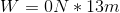# High School Physics : Understanding Work

## Example Questions

### Example Question #1 : Work

A bodybuilder lifts a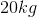weight upward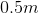, and then returns it to its original position, all with a constant speed. What is the net work done on the weight?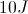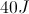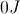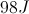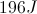Explanation:

Work is a vector quantity equal to the change in energy in a given direction. Our net work will be equal to product of force and displacement.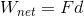We can write this equation in terms of Newton's second law to incorporate our given values.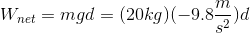During its motion the weight travels a distance ofupward, and thendownward. Its total distance will be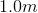, however, we are looking for its displacement. The initial height and final height are equal, making the displacement equal to zero.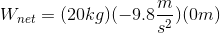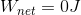The total work will be zero because there was no net displacement.

### Example Question #1 : Understanding Work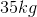crate slides along the floor for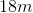. What other piece of information do we need to find the work done?

Potential energy

Time

Velocity

Power

Acceleration

Acceleration

Explanation:

The formula for work is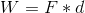, or work equals force times distance.

Since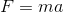, you can expand the work formula a little bit to see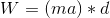.

The problem gives us the mass and the distance traveled, but not the acceleration. Given the acceleration, along with the mass and distance, we would be able to calculate the work.

### Example Question #3 : Work

Miguel pushes a crate with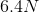of force. What other piece of information do we need to find the work he does?

Time

Velocity

Energy

Distance

Acceleration

Distance

Explanation:

The formula for work is, or work equals force times distance.

The problem gives us the mass, but is missing the distance. Given the distance, we would be able to calculate the work.

### Example Question #4 : Work

Which of the following is an example of work?

A man sits in a chair.

A car drives along the road at a constant speed.

A weightlifter lifts a heavy barbell above his head.

A skier skies down a frictionless hill with no loss in kinetic energy.

A student types numerous essays for class.

A weightlifter lifts a heavy barbell above his head.

Explanation:

Work is a force times a distance orOnly one of these has a force times a distance: the weightlifter lifting a weight above his head.

The car moving at a constant velocity has no acceleration and therefore no force.

Typing essays does not require a force times a distance (though it sure feels like work!).

If the skier has no loss in energy, then no work was done, as work is also the measurement of the change in energy.

The man sitting in a chair has a constant velocity of zero, no acceleration, and therefore no force.

### Example Question #5 : Work

Which of these is a correct formula for work?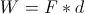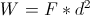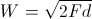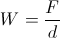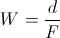Explanation:

Work is produced when a force is used to create a change in distance. The amount of work is given by the product of the distance traveled and the force applied.### Example Question #6 : Work

Michael pushes a box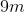across the floor. If the box moves with a constant velocity, what is the total work done?We need to know the force used to find the work

We need to know the time to find the work

We need to know the mass of the box to find the work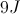Explanation:

Works is equal to force times distance:Since the box moves with a constant velocity, it has no acceleration. Remember that, so if there is no acceleration, then there must be no net force.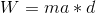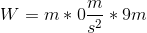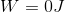If there is no net force, then there is also no work.

### Example Question #7 : Work

Rodrigo pushes a sofa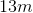down a long hallway. If the couch moves with a constant velocity, how much work does Rodrigo do?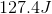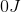We need to know the coefficient of friction of the sofa on the floor to solve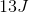We need to know the mass of the sofa to solveExplanation:

Work is the product of a net force over a given distance.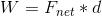In order for there to be work, there must be a net force applied and the object must have a non-zero displacement. In this question we are given the displacement, but we have to solve for the force.

The question only tells us that the couch moves with constant velocity. This tells us that acceleration is zero. If acceleration is zero, then force must also be zero according to Newton's second law.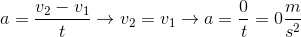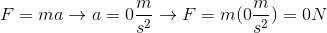If the force is zero, then the work is also zero.